# NCERT Class X: Mathematics-Exercise Solutions

### Chapter 06-Triangles-Exercise Solutions

Class 10- Chapter 6 – Triangles
NCERT Book Page Number-122
Q.1. Fill in the blanks using correct word given in the brackets:−
(i) All circles are __________. (congruent, similar)
(ii) All squares are __________. (similar, congruent)
(iii) All __________ triangles are similar. (isosceles, equilateral)
(iv) Two polygons of the same number of sides are similar, if (a) their corresponding angles are __________ and (b) their corresponding sides are __________. (equal, proportional)
(i) Similar
(ii) Similar
(iii) Equilateral
(iv) (a) Equal
(b) Proportional
Q.2. Give two different examples of pair of
(i) Similar figures
(ii)Non-similar figures
(i) Two equilateral triangles with sides 1 cm and 2 cm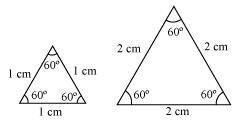Two squares with sides 1 cm and 2 cm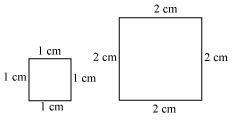(ii) Trapezium and square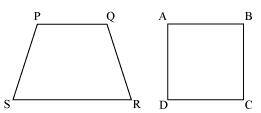(iii)Triangle and parallelogram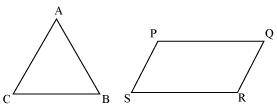Q.3. State whether the following quadrilaterals are similar or not: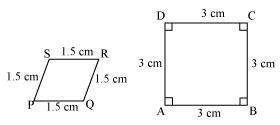Answer: Quadrilateral PQRS and ABCD are not similar as their corresponding sides are proportional, i.e. 1:2, but their corresponding angles are not equal.
NCERT Book Page Number-128
Q.1. In figure (i) and (ii), DE || BC. Find EC in (i) and AD in (ii).
(i)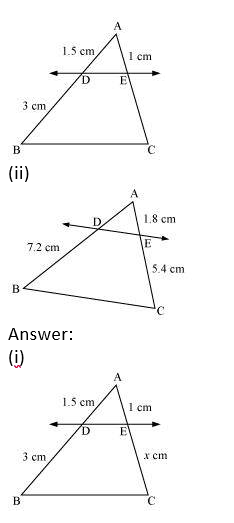Let EC = x cm
It is given that DE || BC.
By using basic proportionality theorem, we obtain
Fraction = $\frac{AB}{DB} = \frac{AE}{EC}$
Fraction = $\frac{1.5}{3} = \frac{1}{x}$
x = $\frac{3 × 1}{1.5}$
x = 2
 EC = 2 cm

(ii)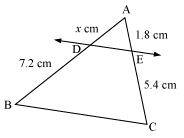It is given that DE || BC.
By using basic proportionality theorem, we obtain

Fraction = $\frac{AD}{DB} = \frac{AE}{EC}$
Fraction = $\frac{x}{7.2} = \frac{1.8}{5.4}$
x = $\frac{1.8 × 7.2}{5.4}$
x = 2.4

Q.2. E and F are points on the sides PQ and PR respectively of a ΔPQR. For each of the following cases, state whether EF || QR.
(i) PE = 3.9 cm, EQ = 3 cm, PF = 3.6 cm and FR = 2.4 cm
(ii) PE = 4 cm, QE = 4.5 cm, PF = 8 cm and RF = 9 cm
(iii)PQ = 1.28 cm, PR = 2.56 cm, PE = 0.18 cm and PF = 0.63 cm
(i)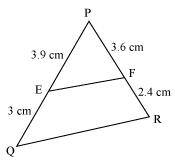Given that, PE = 3.9 cm, EQ = 3 cm, PF = 3.6 cm, FR = 2.4 cm
Fraction = $\frac{PQ}{EQ} = \frac{3.9}{3} =1.3$
Fraction = $\frac{PF}{FR} = \frac{3.6}{2.4} = 1.5$
Hence, $\frac{PE}{EQ} \neq \frac{PF}{FR}$
Therefore, EF is not parallel to QR.

(ii)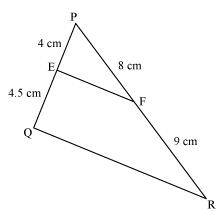PE = 4 cm, QE = 4.5 cm, PF = 8 cm, RF = 9 cm

$\frac{PE}{EQ} = \frac{4}{4.5} = \frac{8}{9}$
$\frac{PF}{FR} = \frac{8}{9}$
Hence, $\frac{PE}{EQ} = \frac{PF}{FR}$
Therefore, EF is parallel to QR.

(iii)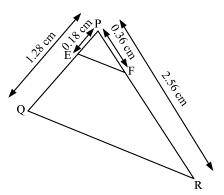PQ = 1.28 cm, PR = 2.56 cm, PE = 0.18 cm, PF = 0.36 cm
$\frac{PE}{EQ} = \frac{0.18}{1.28} = \frac{18}{128} = \frac{9}{64}$
$\frac{PF}{PR} = \frac{0.36}{2.56} = \frac{9}{64}$
Hence, $\frac{PE}{PQ} = \frac{PF}{PR}$
Therefore, EF is parallel to QR.

Q.3. In the following figure, if LM || CB and LN || CD, prove that
$\frac{AM}{AB} = \frac{AN}{AD}$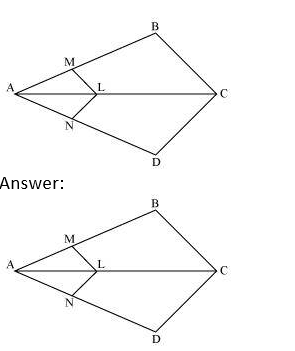In the given figure, LM || CB
By using basic proportionality theorem, we obtain

$\frac{AM}{AB} = \frac{AL}{AC} —————(i)$
Similarly, LN||CD
$\frac{AN}{AD} = \frac{AL}{AC} ————(ii)$
From (i) and (ii), we obtain
$\frac{AM}{AB} = \frac{AN}{AD}$

Q.4. In the following figure, DE || AC and DF || AE. Prove that
$\frac{BF}{FE} = \frac{BE}{EC}$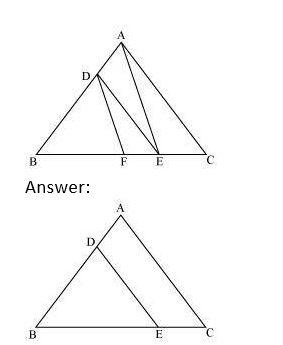In ΔABC, DE || AC
$\therefore \frac{BD}{DA} = \frac{BE}{EC}$ (Basic Property Theorem)——-(i)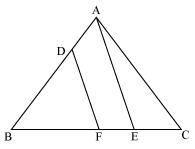$in \triangle BAE, DF \parallel AE$
$\therefore \frac{BD}{DA} = \frac{BF}{FE}$ (Basic Property Theorem)—–(ii)
From (i) and (ii), we have
$\frac{BE}{EC} = \frac{BF}{FE}$
NCERT Book Page Number-129
Q.5. In the following figure, DE || OQ and DF || OR, show that EF || QR.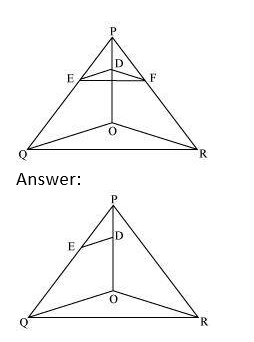In Δ POQ, DE || OQ
$\therefore \frac{PE}{EQ} = \frac{PD}{DO}$(Basic proportionality theorem)—————–(i)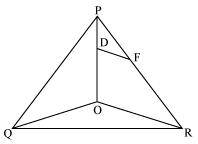$In\triangle POR, DF\parallel OR$
$\frac{PF}{FR}= \frac{PD}{DO}$ (Basic proportionality theorem)———–(ii)
From (i) and (ii), we obtain
$frac{PE}{EQ}= \frac{PF}{FR}$
$\therefore EF\parallel QR$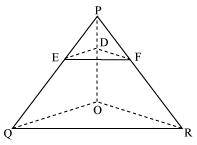Q.6. In the following figure, A, B and C are points on OP, OQ and OR respectively such that AB || PQ and AC || PR. Show that BC || QR.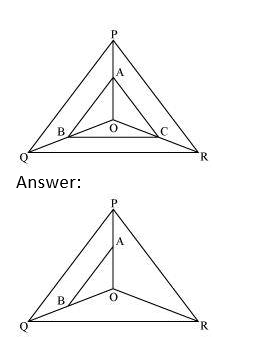In Δ POQ, AB || PQ
$\frac{OA}{AP}= \frac{OB}{BQ}$ (Basic proportionality theorem)———–(i)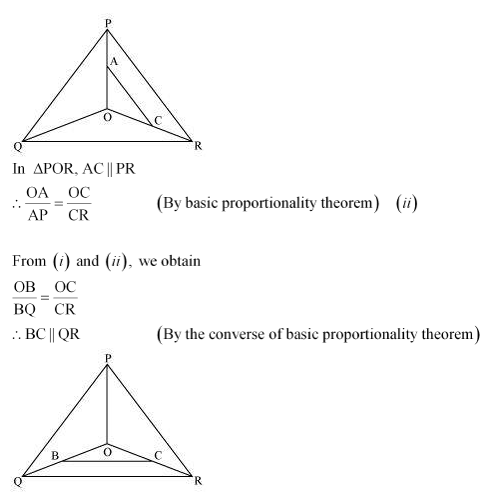Q.7. Using Basic proportionality theorem, prove that a line drawn through the mid-points of one side of a triangle parallel to another side bisects the third side. (Recall that you have proved it in Class IX).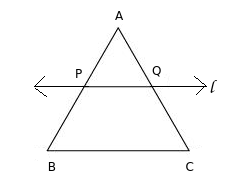Consider the given figure in which l is a line drawn through the mid-point P of line segment AB meeting AC at Q, such that PQ ||BC.
By using basic proportionality theorem, we obtain
$\frac{AQ}{QC} = \frac{AP}{PB}$
$\frac{AQ}{QC} = \frac{1}{1}$ (P is the mid-point of AB) $\therefore$ AP=PB
$\implies AQ = QC$
Or, Q is the mid-point of AC.
Q.8. Using Converse of basic proportionality theorem, prove that the line joining the mid-points of any two sides of a triangle is parallel to the third side. (Recall that you have done it in Class IX).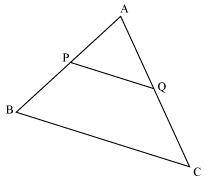Consider the given figure in which PQ is a line segment joining the mid-points P and Q of line AB and AC respectively.
i.e., AP = PB and AQ = QC
It can be observed that
$\frac{AP}{PB} = \frac{1}{1}$
and $\frac{AQ}{QC} = \frac{1}{1}$
$\therefore \frac{AP}{PB} = \frac{AQ}{QC}$
Hence, by using basic proportionality theorem, we obtain
$PQ \parallel BC$

Q.9. ABCD is a trapezium in which AB || DC and its diagonals intersect each other at the point O. Show that $\frac{AO}{BO} = \frac{CO}{DO}$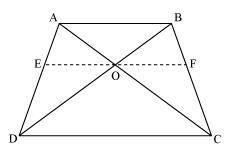Draw a line EF through point O, such that EF||CD
By using basic proportionality theorem, we obtain $\fraction{AE}{ED}=\fraction{AO}{OC}$ ———(1)
In ΔABD, OE ||AB
So, by using basic proportionality theorem, we obtain
$\frac{ED}{AE}=\frac{BO}{OD}$
Or, $\frac{AE}{ED}=\frac{AO}{OD}$ ———(2)
From equations (1) and (2), we obtain
$\frac{AO}{OC}=\frac{BO}{OD}$
Or, $\frac{AO}{BO}=\frac{OC}{OD}$
Q.10. The diagonals of a quadrilateral ABCD intersect each other at the point O such that $\frac{AO}{BO}=\frac{CO}{DO}$ Show that ABCD is a trapezium.
Answer: Let us consider the following figure for the given question.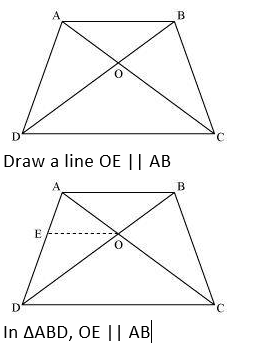By using basic proportionality theorem, we obtain
$\frac{AE}{ED} = \frac{BO}{OD}$—————-(1)
However, it is given that
$\frac{AO}{OC} = \frac{OB}{OD}$—————-(2)
From equation (1) and (2) we obtain
$\frac{AE}{ED} = \frac{AO}{OC}$
⇒ EO || DC [By the converse of basic proportionality theorem]
⇒ AB || OE || DC
⇒ AB || CD
∴ ABCD is a trapezium.
NCERT Book Page Number-138
Q.1. State which pairs of triangles in the following figure are similar? Write the similarity criterion used by you for answering the question and also write the pairs of similar triangles in the symbolic form:
(i)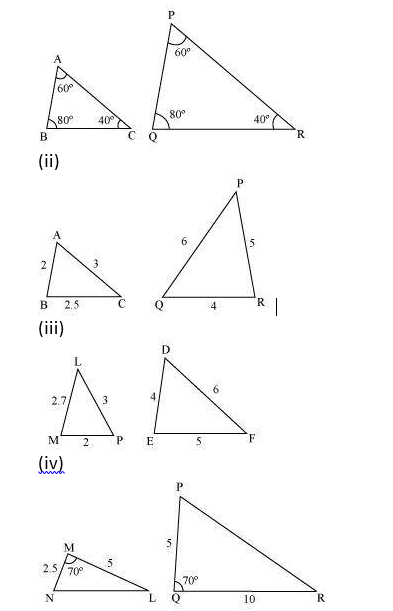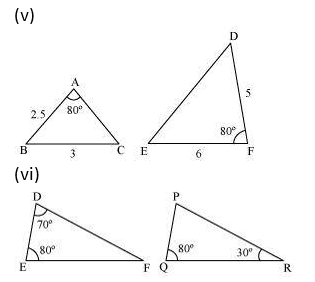(i) $\angle A = \angle P = 60°$
$\angle B = \angle Q = 80°$
$\angle C = \ angle R = 40°$
Therefore, $\triangle ABC ∼ \triangle PQR$ [By AAA similarity criterion]
$\frac{AB}{QR}=\frac{BC}{RP}=\frac{CA}{PQ}$
(ii) $\therefore \triangle ABC~ \triangle QRP$ [By SSS Simililarity criterian]
(iii)The given triangles are not similar as the corresponding sides are not proportional.
(iv) In a triangle MNL and triangle QPR, we observe that,

$\frac{MN}{QP} = \frac{ML}{QR}$
And ∠M = ∠Q = 70°
∴∆MNL ~ ∆QPR By SAS similarity criterion
(v)The given triangles are not similar as the corresponding sides are not proportional.
(vi) In ΔDEF,
∠D +∠E +∠F = 180º
(Sum of the measures of the angles of a triangle is 180º.)
70º + 80º +∠F = 180º
∠F = 30º
Similarly, in ΔPQR,
∠P +∠Q +∠R = 180º
(Sum of the measures of the angles of a triangle is 180º.)
∠P + 80º +30º = 180º
∠P = 70º
In ΔDEF and ΔPQR,
∠D = ∠P (Each 70°)
∠E = ∠Q (Each 80°)
∠F = ∠R (Each 30°)
∴ ΔDEF ∼ ΔPQR [By AAA similarity criterion]
NCERT Book Page Number-139
Q.2. In the following figure, ΔODC ∼ ΔOBA, ∠BOC = 125° and ∠CDO = 70°. Find ∠DOC, ∠DCO and ∠OAB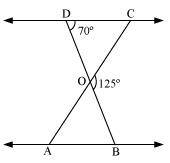DOB is a straight line.
∴ ∠DOC + ∠COB = 180°
⇒ ∠DOC = 180° − 125°
= 55°
In ΔDOC,
∠DCO + ∠CDO + ∠DOC = 180°
(Sum of the measures of the angles of a triangle is 180º.)
⇒ ∠DCO + 70º + 55º = 180°
⇒ ∠DCO = 55°
It is given that ΔODC ∼ ΔOBA.
∴ ∠OAB = ∠ OCD [Corresponding angles are equal in similar triangles.]
⇒ ∠OAB = 55°
Q.3. Diagonals AC and BD of a trapezium ABCD with AB || DC intersect each other at the point O. Using a similarity criterion for two triangles, show that $\frac {AO}{OC} = \frac{OB}{OD}$.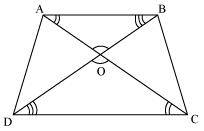In ΔDOC and ΔBOA,
∠CDO = ∠ABO [Alternate interior angles as AB || CD]
∠DCO = ∠BAO [Alternate interior angles as AB || CD]
∠DOC = ∠BOA [Vertically opposite angles]
∴ ΔDOC ∼ ΔBOA [AAA similarity criterion]
$\therefore \frac{DO}{BO}=\frac{OC}{OA}$[Corresponding sides are proportional]
$\implies \frac{OA}{OC}=\frac{OB}{OD}$
NCERT Book Page Number-140
Q.4. In the following figure,$\frac{QR}{QS} = \frac{QT}{PR} and <1 = <2.Show that$\triangle PQS ~ \triangle TQR$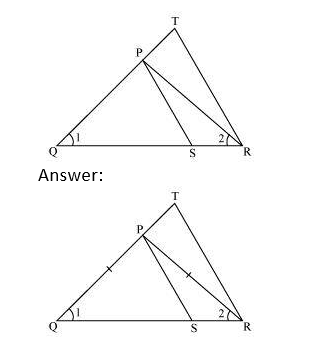In ΔPQR, ∠PQR = ∠PRQ ∴ PQ = PR (i) Given,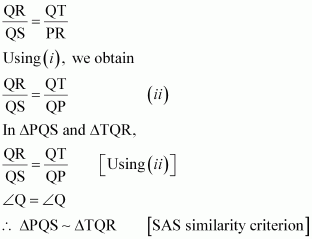Q.5. S and T are point on sides PR and QR of ΔPQR such that ∠P = ∠RTS. Show that ΔRPQ ∼ ΔRTS. Answer: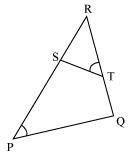In ΔRPQ and ΔRST, ∠RTS = ∠QPS (Given) ∠R = ∠R (Common angle) ∴ ΔRPQ ∼ ΔRTS (By AA similarity criterion) Q.6. In the following figure, if ΔABE ≅ ΔACD, show that ΔADE ∼ ΔABC.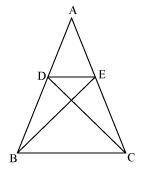Answer: It is given that ΔABE ≅ ΔACD. ∴ AB = AC [By CPCT] ——————–(1) And, AD = AE [By CPCT]—————- (2) In ΔADE and ΔABC,$\frac{AD}{AB}=\frac{AE}{AC}$[Dividing equation (2) by (1)] ∠A = ∠A [Common angle] ∴ ΔADE ∼ ΔABC [By SAS similarity criterion] Q.7. In the following figure, altitudes AD and CE of ΔABC intersect each other at the point P. Show that: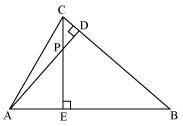(i) ΔAEP ∼ ΔCDP (ii) ΔABD ∼ ΔCBE (iii) ΔAEP ∼ ΔADB (v) ΔPDC ∼ ΔBEC Answer: (i)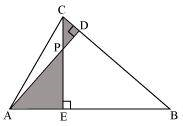In ΔAEP and ΔCDP, ∠AEP = ∠CDP (Each 90°) ∠APE = ∠CPD (Vertically opposite angles) Hence, by using AA similarity criterion, ΔAEP ∼ ΔCDP (ii)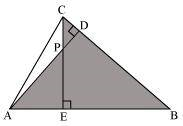In ΔABD and ΔCBE, ∠ADB = ∠CEB (Each 90°) ∠ABD = ∠CBE (Common) Hence, by using AA similarity criterion, ΔABD ∼ ΔCBE (iii)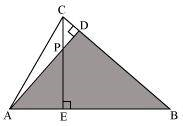In ΔAEP and ΔADB, ∠AEP = ∠ADB (Each 90°) ∠PAE = ∠DAB (Common) Hence, by using AA similarity criterion, ΔAEP ∼ ΔADB (iv)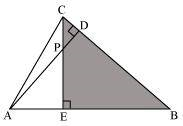In ΔPDC and ΔBEC, ∠PDC = ∠BEC (Each 90°) ∠PCD = ∠BCE (Common angle) Hence, by using AA similarity criterion, ΔPDC ∼ ΔBEC Video Solution Q.8. E is a point on the side AD produced of a parallelogram ABCD and BE intersects CD at F. Show that ΔABE ∼ ΔCFB Answer: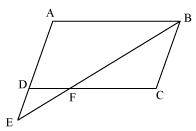In ΔABE and ΔCFB, ∠A = ∠C (Opposite angles of a parallelogram) ∠AEB = ∠CBF (Alternate interior angles as AE || BC) ∴ ΔABE ∼ ΔCFB (By AA similarity criterion) Q.9. In the following figure, ABC and AMP are two right triangles, right angled at B and M respectively, prove that: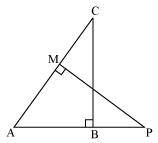(i) ΔABC ∼ ΔAMP (ii)$\frac{CA}{PA} = \frac{BC}{MP}$Answer: In ΔABC and ΔAMP, ∠ABC = ∠AMP (Each 90°) ∠A = ∠A (Common) ∴ ΔABC ∼ ΔAMP (By AA similarity criterion)$\implies \frac{CA}{PA}=\frac{BC}{MP}$(Corresponding sides of similar triangles are proportional) Q.10. CD and GH are respectively the bisectors of ∠ACB and ∠EGF such that D and H lie on sides AB and FE of ΔABC and ΔEFG respectively. If ΔABC ∼ ΔFEG, Show that: (i)$\frac{CD}{GH}=\frac{AC}{FG}$(ii) ΔDCB ∼ ΔHGE (iii) ΔDCA ∼ ΔHGF Answer: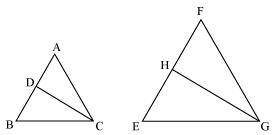It is given that ΔABC ∼ ΔFEG. ∴ ∠A = ∠F, ∠B = ∠E, and ∠ACB = ∠FGE ∠ACB = ∠FGE ∴ ∠ACD = ∠FGH (Angle bisector) And, ∠DCB = ∠HGE (Angle bisector) In ΔACD and ΔFGH, ∠A = ∠F (Proved above) ∠ACD = ∠FGH (Proved above) ∴ ΔACD ∼ ΔFGH (By AA similarity criterion)$\implies \frac{CD}{GH}=\frac{AC}{FG}$In ΔDCB and ΔHGE, ∠DCB = ∠HGE (Proved above) ∠B = ∠E (Proved above) ∴ ΔDCB ∼ ΔHGE (By AA similarity criterion) In ΔDCA and ΔHGF, ∠ACD = ∠FGH (Proved above) ∠A = ∠F (Proved above) ∴ ΔDCA ∼ ΔHGF (By AA similarity criterion) NCERT Book Page Number-141 Q.11. In the following figure, E is a point on side CB produced of an isosceles triangle ABC with AB = AC. If AD ⊥ BC and EF ⊥ AC, prove that ΔABD ∼ ΔECF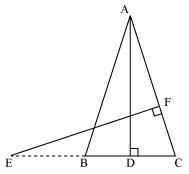Answer: It is given that ABC is an isosceles triangle. ∴ AB = AC ⇒ ∠ABD = ∠ECF In ΔABD and ΔECF, ∠ADB = ∠EFC (Each 90°) ∠ABD = ∠ECF (Proved above) ∴ ΔABD ∼ ΔECF (By using AA similarity criterion) Q.12. Sides AB and BC and median AD of a triangle ABC are respectively proportional to sides PQ and QR and median PM of ΔPQR (see the given figure). Show that ΔABC ~ ΔPQR. Answer: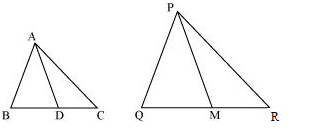Median divides the opposite side.$\therefore BD = \frac{BC}{2} and QM =\frac{QR}{2}$Given that,$\frac{AB}{PQ}=\frac{BC}{QR}=\frac{AD}{PM}\implies \frac{AB}{PQ}= \frac{1/2 BC}{1/2 QR}=\frac{AD}{PM}\implies \frac{AB}{PQ}= \frac{BD}{QM}=\frac{AD}{PM}$In ΔABD and ΔPQM,$\frac{AB}{PQ}=\frac{BD}{QM}=\frac{AD}{PM}$(Proved above) ∴ ΔABD ∼ ΔPQM (By SSS similarity criterion) ⇒ ∠ABD = ∠PQM (Corresponding angles of similar triangles) In ΔABC and ΔPQR, ∠ABD = ∠PQM (Proved above)$\frac{AB}{PQ}=\frac{BC}{QR}$∴ ΔABC ∼ ΔPQR (By SAS similarity criterion) Q.13. D is a point on the side BC of a triangle ABC such that ∠ADC = ∠BAC. Show that$CA^{2}$= CB.CD$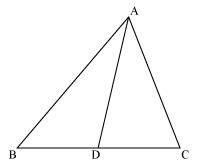$\therefore \frac{CA}{CB}=\frac{CD}{CA}$
$\implies CA^{2}= CB × CD$
Q.14. Sides AB and AC and median AD of a triangle ABC are respectively proportional to sides PQ and PR and median PM of another triangle PQR. Show that $\triangle ABC ~ \triangle PQR$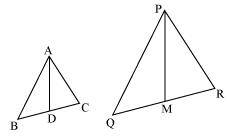$\frac{AB}{PQ}=\frac{AC}{PR}=\frac{AD}{PM}$